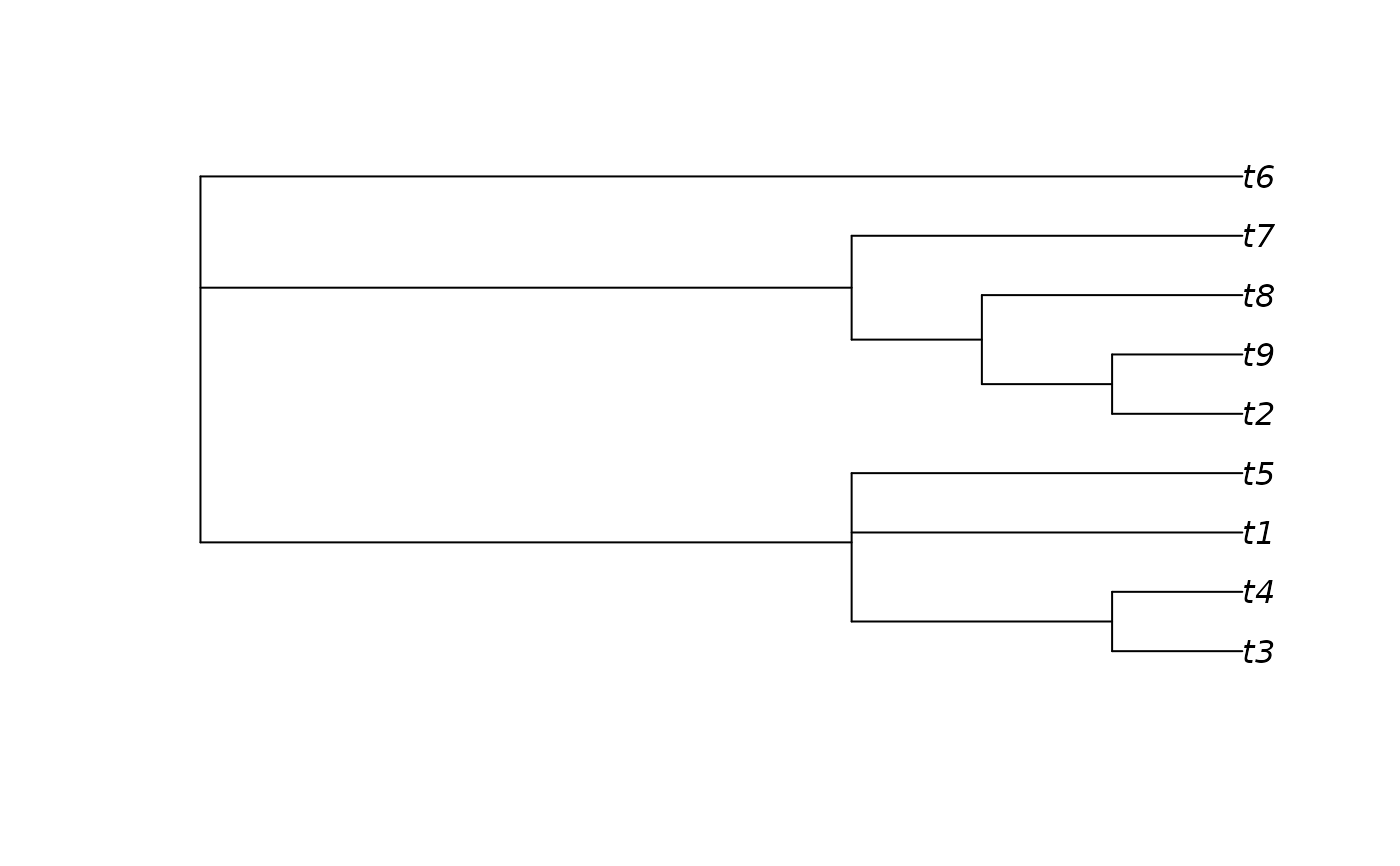SplitFrequency() provides a simple way to count the number of times that bipartition splits, as defined by a reference tree, occur in a forest of trees. May be used to calculate edge ("node") support for majority consensus or bootstrap trees.

SplitFrequency(reference, forest)

SplitNumber(tips, tree, tipIndex, powersOf2)

ForestSplits(forest, powersOf2)

TreeSplits(tree)

## Arguments

reference

A tree of class phylo, a Splits object.

forest

a list of trees of class phylo, or a multiPhylo object; or a Splits object. See vignette for possible methods of loading trees into R.

tips

Integer vector specifying the tips of the tree within the chosen split.

tree

A tree of class phylo.

tipIndex

Character vector of tip names, in a fixed order.

powersOf2

Integer vector of same length as tipIndex, specifying a power of 2 to be associated with each tip in turn.

## Value

SplitFrequency() returns the number of trees in forest that contain each split in reference. If reference is a tree of class phylo, then the sequence will correspond to the order of nodes (use ape::nodelabels() to view). Note that the three nodes at the root of the tree correspond to a single split; see the example for how these might be plotted on a tree.

## Details

If multiple calculations are required, some time can be saved by using the constituent functions (see examples)

## Functions

• SplitNumber: Assign a unique integer to each split

• ForestSplits: Frequency of splits in a given forest of trees

• TreeSplits: Deprecated. Listed the splits in a given tree. Use as.Splits instead.

Other Splits operations: LabelSplits(), NSplits(), NTip(), PolarizeSplits(), SplitsInBinaryTree(), Splits, TipLabels(), TipsInSplits(), match.Splits, xor()

## Author

Martin R. Smith (martin.smith@durham.ac.uk)

## Examples

# An example forest of 100 trees, some identical
forest <- as.phylo(c(1, rep(10, 79), rep(100, 15), rep(1000, 5)), nTip = 9)

# Generate an 80% consensus tree
cons <- ape::consensus(forest, p = 0.8)
plot(cons)

splitFreqs <- SplitFrequency(cons, forest)
LabelSplits(cons, splitFreqs, unit = '%',
col = SupportColor(splitFreqs / 100),
frame = 'none', pos = 3L)